# list assignment

## Partial List Coloring ★★★

Letbe a simple graph, and for every list assignmentlet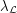be the maximum number of vertices ofwhich are colorable with respect to. Define, where the minimum is taken over all list assignmentswithfor all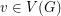.

Conjecture    Letbe a graph with list chromatic numberand. Then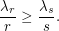Keywords: list assignment; list coloring

## Partial List Coloring ★★★

Author(s): Albertson; Grossman; Haas

Conjecture   Letbe a simple graph withvertices and list chromatic number. Suppose that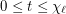and each vertex ofis assigned a list ofcolors. Then at least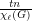vertices ofcan be colored from these lists.

Keywords: list assignment; list coloring